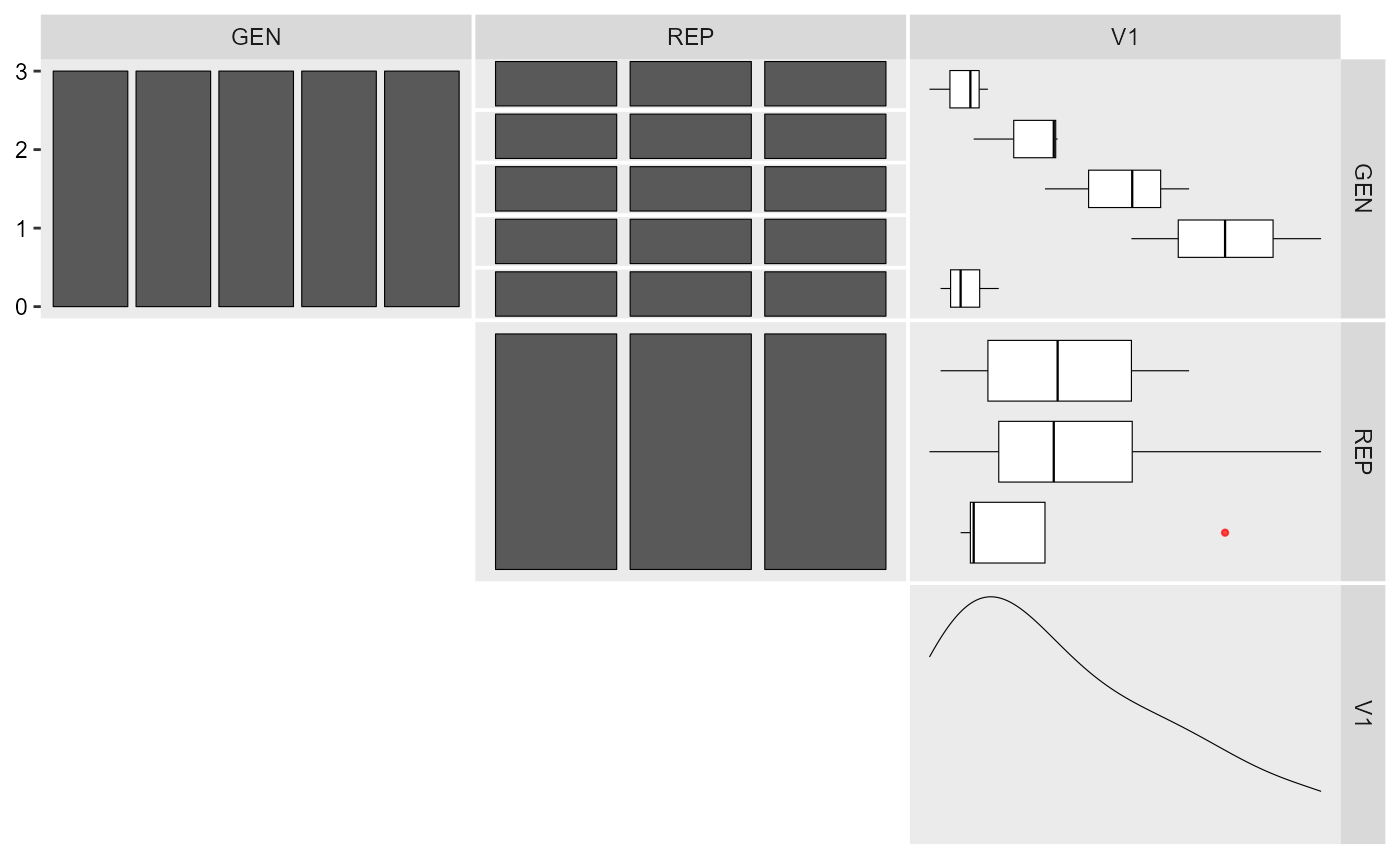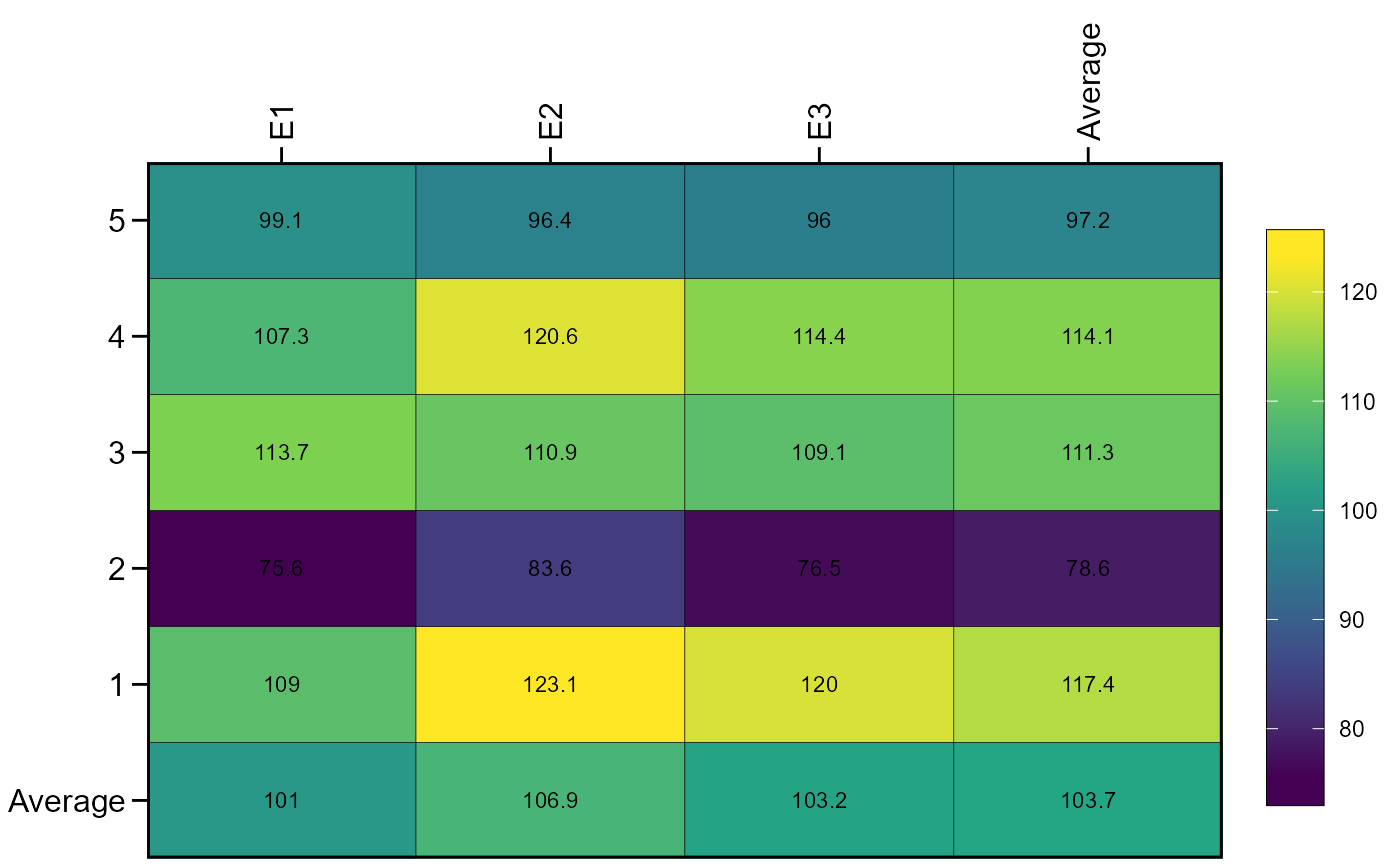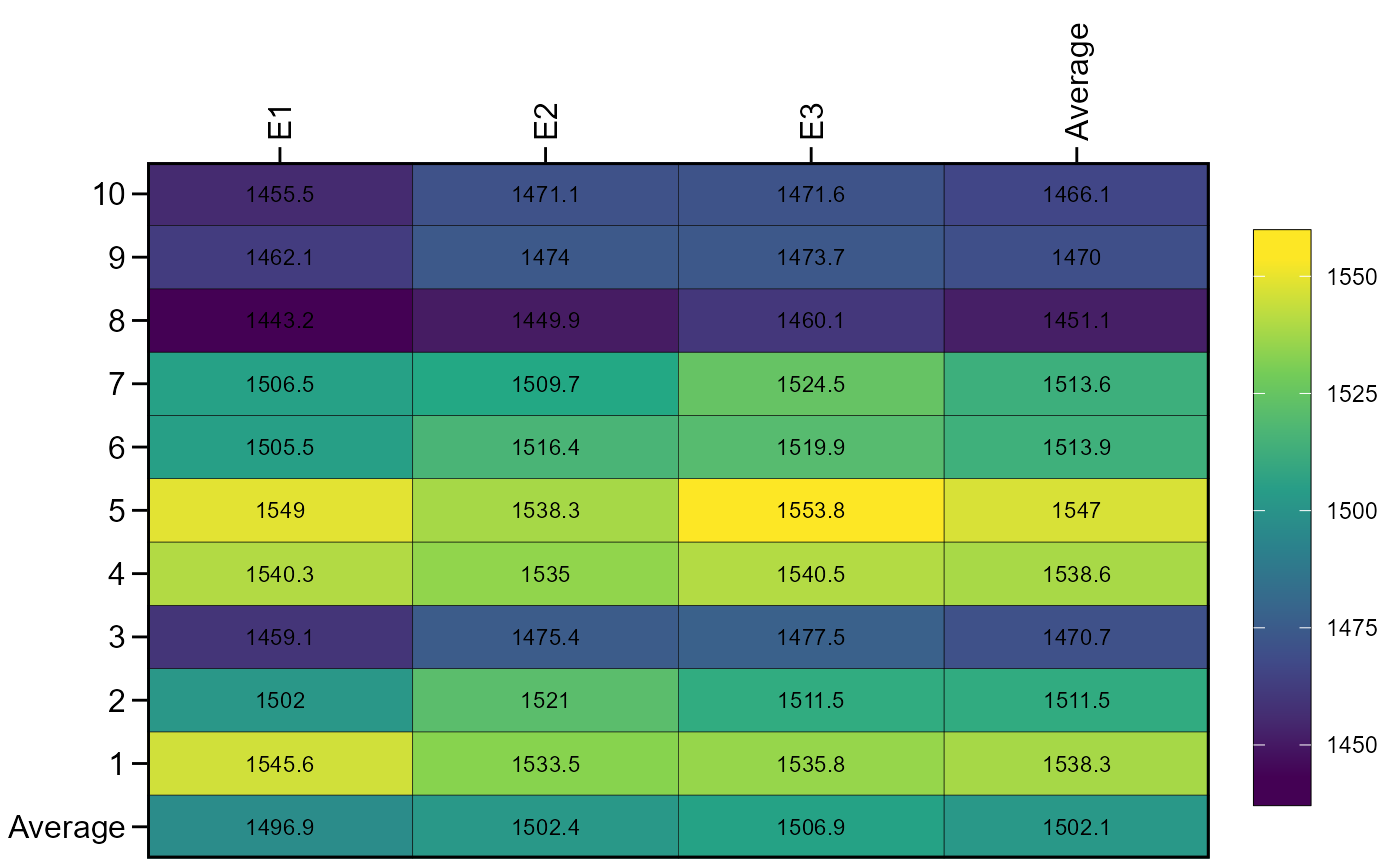• g_simula() simulate replicated genotype data.

• ge_simula() simulate replicated genotype-environment data.

## Usage

ge_simula(
ngen,
nenv,
nrep,
nvars = 1,
gen_eff = 20,
env_eff = 15,
rep_eff = 5,
ge_eff = 10,
res_eff = 5,
intercept = 100,
seed = NULL
)

g_simula(
ngen,
nrep,
nvars = 1,
gen_eff = 20,
rep_eff = 5,
res_eff = 5,
intercept = 100,
seed = NULL
)

## Arguments

ngen

The number of genotypes.

nenv

The number of environments.

nrep

The number of replications.

nvars

The number of traits.

gen_eff

The genotype effect.

env_eff

The environment effect

rep_eff

The replication effect

ge_eff

The genotype-environment interaction effect.

res_eff

The residual effect. The effect is sampled from a normal distribution with zero mean and standard deviation equal to res_eff. Be sure to change res_eff when changin the intercept scale.

intercept

The intercept.

seed

The seed.

## Value

A data frame with the simulated traits

## Details

The functions simulate genotype or genotype-environment data given a desired number of genotypes, environments and effects. All effects are sampled from an uniform distribution. For example, given 10 genotypes, and gen_eff = 30, the genotype effects will be sampled as runif(10, min = -30, max = 30). Use the argument seed to ensure reproducibility. If more than one trait is used (nvars > 1), the effects and seed can be passed as a numeric vector. Single numeric values will be recycled with a warning when more than one trait is used.

## Author

Tiago Olivoto tiagoolivoto@gmail.com

## Examples

# \donttest{
library(metan)
# Genotype data (5 genotypes and 3 replicates)
gen_data <-
g_simula(ngen = 5,
nrep = 3,
seed = 1)
gen_data
#> # A tibble: 15 × 3
#>    GEN   REP      V1
#>    <fct> <fct> <dbl>
#>  1 H1    B1     96.3
#>  2 H1    B2     91.0
#>  3 H1    B3     94.7
#>  4 H2    B1    103.
#>  5 H2    B2    102.
#>  6 H2    B3     95.0
#>  7 H3    B1    114.
#>  8 H3    B2    109.
#>  9 H3    B3    101.
#> 10 H4    B1    109.
#> 11 H4    B2    126.
#> 12 H4    B3    118.
#> 13 H5    B1     92.0
#> 14 H5    B2     97.2
#> 15 H5    B3     93.8
inspect(gen_data, plot = TRUE)
#> # A tibble: 3 × 10
#>   Variable Class   Missing Levels Valid_n   Min Median   Max Outlier Text
#>   <chr>    <chr>   <chr>   <chr>    <int> <dbl>  <dbl> <dbl>   <dbl> <lgl>
#> 1 GEN      factor  No      5           15  NA      NA    NA       NA NA
#> 2 REP      factor  No      3           15  NA      NA    NA       NA NA
#> 3 V1       numeric No      -           15  91.0   101.  126.       0 NA
#> Warning: Expected three or more factor variables. The data has only 2.aov(V1 ~ GEN + REP, data = gen_data) %>% anova()
#> Analysis of Variance Table
#>
#> Response: V1
#>           Df  Sum Sq Mean Sq F value   Pr(>F)
#> GEN        4 1242.03 310.508 10.1917 0.003146 **
#> REP        2   55.60  27.798  0.9124 0.439611
#> Residuals  8  243.74  30.467
#> ---
#> Signif. codes:  0 '***' 0.001 '**' 0.01 '*' 0.05 '.' 0.1 ' ' 1

# Genotype-environment data
# 5 genotypes, 3 environments, 4 replicates and 2 traits
df <-
ge_simula(ngen = 5,
nenv = 3,
nrep = 4,
nvars = 2,
seed = 1)
#> Warning: 'gen_eff = 20' recycled for all the 2 traits.
#> Warning: 'env_eff = 15' recycled for all the 2 traits.
#> Warning: 'rep_eff = 5' recycled for all the 2 traits.
#> Warning: 'ge_eff = 10' recycled for all the 2 traits.
#> Warning: 'res_eff = 5' recycled for all the 2 traits.
#> Warning: 'intercept = 100' recycled for all the 2 traits.
#> Warning: 'seed = 1' recycled for all the 2 traits.
ge_plot(df, ENV, GEN, V1)aov(V1 ~ ENV*GEN + ENV/REP, data = df) %>% anova()
#> Analysis of Variance Table
#>
#> Response: V1
#>           Df  Sum Sq Mean Sq  F value    Pr(>F)
#> ENV        2   363.7  181.83  13.0240 5.551e-05 ***
#> GEN        4 12319.5 3079.87 220.6002 < 2.2e-16 ***
#> ENV:GEN    8   643.9   80.48   5.7647 9.551e-05 ***
#> ENV:REP    9   426.2   47.36   3.3920   0.00415 **
#> Residuals 36   502.6   13.96
#> ---
#> Signif. codes:  0 '***' 0.001 '**' 0.01 '*' 0.05 '.' 0.1 ' ' 1

# Change genotype effect (trait 1 with fewer differences among genotypes)
# Define different intercepts for the two traits
df2 <-
ge_simula(ngen = 10,
nenv = 3,
nrep = 4,
nvars = 2,
gen_eff = c(1, 50),
intercept = c(80, 1500),
seed = 1)
#> Warning: 'env_eff = 15' recycled for all the 2 traits.
#> Warning: 'rep_eff = 5' recycled for all the 2 traits.
#> Warning: 'ge_eff = 10' recycled for all the 2 traits.
#> Warning: 'res_eff = 5' recycled for all the 2 traits.
#> Warning: 'seed = 1' recycled for all the 2 traits.
ge_plot(df2, ENV, GEN, V2)# }